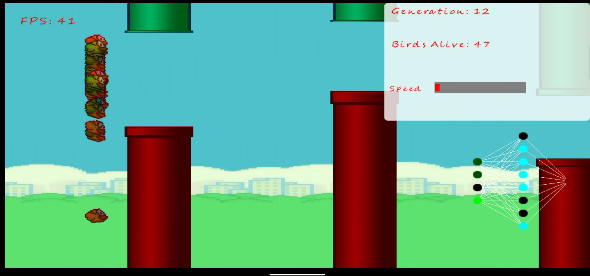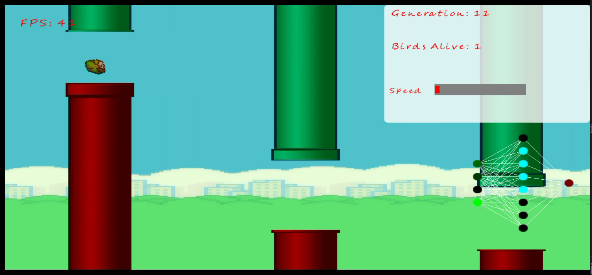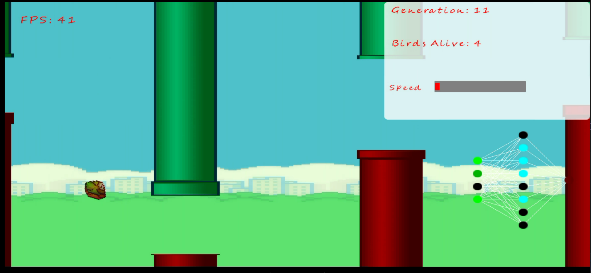# Machine Learning

This is an flappy bird implementation using Artificial Neural Network and the Genetics Algorithm(Neural Evolution). The genetics algorithm is used to train the network instead of us dictating the output using traditional labels which would be impossible for this type of problem.

## Architecture

In this simulation there are there Layers in the neuron Network:-

• 4 Input Neurons

``````1. The position of the bird in the Y Axis.
2. The center position of the gap.
3. Difference between the Upper pillar and bird Y position.
4. Difference between the Lower pillaer and the bird Y position.
``````
• 8 Hidden Neurons

• 1 Output Neuron

``````1. If output is greater than 0.5 then fly upwards.
``````

## Procedure

• create a population with random weights.
• Define a fitness function:- can be the maximum distance travelled by the bird along the X axis.
• Sort the population based on the fitness or score.
• Selection:- Select 70%, 50 , 30% etc. of the best birds (Natural selection only favours the strong,the weak will perish).
• CrossOver:- After selection duplicate the birds genes (the weighs in the neural network including the bias values).
• Mutation:- – Finally mutate the children to create variation. NBIn the mutation function instead of assigning new random weights just modify the weight slightly to give better results.

```     fun  mutate(rate:Float){
for (i in 0 until rows){
for(j in 0 until cols){
if(Random.nextFloat()<=rate)
// numbers closer to the mean are more likely to be selected better than Random.nextDouble()
data[i][j]+=(java.util.Random().nextGaussian()*2.0 -1)*0.1
}
}
}   ```
• Repeat.

## ScreenShots

• I marked the birds with random gradients to see which birds passed their genes to the next generation.View Github